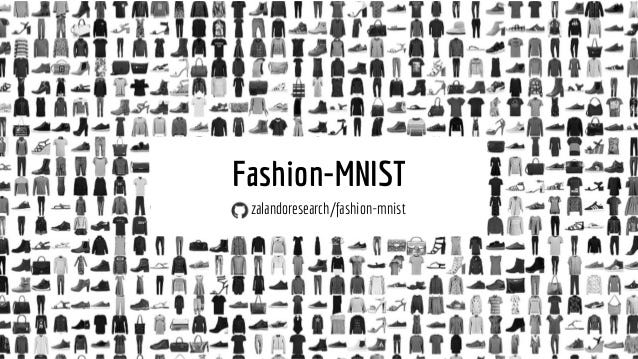# [Deep Learning Lab] Episode-1: Fashion-MNIST

Let’s “Deep Learning Lab” begins!

This is the first episode of “Deep Learning Lab” story series which contains my individual works for deep learning with different cases.

The dataset for the first episode that I would like to work on is MNIST dataset -not surprizingly-. However, it is not MNIST handwritten digit database as first come to your mind, but MNIST-like fashion product database. Actually, Fashion-MNIST -wow!-.

Fashion-MNIST dataset has been developed by the Zalando Research Team as clothes product database and as an alternative to the original MNIST handwritten digits database. Besides to have the same physical characteristics as the ancestor (the original one), there are 60.000 images for training a model and 10.000 images for evaluating the performance of the model. The most significant reason for picking this dataset is that the vast majority of searches about deep learning on Google may introduce you to the original MNIST, but you are now probably meeting Fashion-MNIST for the first time -don’t you?-.

Let me reference to the real heroes:

Fashion-MNIST: a Novel Image Dataset for Benchmarking Machine Learning Algorithms. Han Xiao, Kashif Rasul, Roland Vollgraf.

https://arxiv.org/abs/1708.07747

Zalando team summarizes why they think that there is the need to create such a dataset for machine learning and deep learning researchers, with these 3 sentences:

1. MNIST is too easy. Convolutional nets can achieve 99.7% on MNIST. Classic machine learning algorithms can also achieve 97% easily.
2. MNIST is overused. In this April 2017 Twitter thread, Google Brain research scientist and deep learning expert Ian Goodfellow calls for people to move away from MNIST.
3. MNIST can not represent modern computer vision tasks.

(Han Xiao et al.)

If I can convince you enough for Fashion-MNIST, let’s start coding.

LET’S GOOOOO!

First, I would like to express my thanks to Fuat from Deep Learning Turkey since he introduced me to Google Colaboratory and helped me to be able to use it.

As you all know, it is not an easy task to own high quality graphics cards which are able to train any deep learning model with. NVIDIA GTX 965M, my lovely GPU, is just an average model although it provides CUDA support. It can train an average (not really deep) model as slow as molasses in January. Thanks to Google Colaboratory, it is completely free to use “Tesla K80 GPU” for the applications such as Tensorflow, Keras and PyTorch via an IPython-based notebook.

I prefer to use Tensorflow and Keras for my works. In the “Deep Learning Lab” series, I would like to choose Keras, which gives you an opportunity to understand how the code works even if you have minimum/no knowledge of the subject -well, not tell a lie; it needs to know a bit about Python, and also to follow the literature-.

Importing the libraries.

`from __future__ import print_functionimport kerasfrom keras.datasets import fashion_mnistfrom keras.models import Sequentialfrom keras.layers import Dense, Dropout, Activation, Flattenfrom keras.layers import Conv2D, MaxPooling2Dfrom keras.utils import print_summaryfrom keras.optimizers import Adamfrom keras.regularizers import l2import os`

Initializing the parameters.

`batch_size = 32 # You can try 64 or 128 if you'd like tonum_classes = 10epochs = 100 # loss function value will be stabilized after 93rd epoch# To save the model:save_dir = os.path.join(os.getcwd(), 'saved_models')model_name = 'keras_fashion_mnist_trained_model.h5'`

Thanks to Keras, we can load the dataset easily.

`(x_train, y_train), (x_test, y_test) = fashion_mnist.load_data()`

We need to reshape the data since the images in the dataset are grayscaled.

`x_train = x_train.reshape(x_train.shape, x_train.shape, x_train.shape, 1)x_test = x_test.reshape(x_test.shape, x_test.shape, x_test.shape, 1)input_shape = (28, 28, 1)`

We also need to convert the labels in the dataset into categorical matrix structure from 1-dim numpy array structure.

`y_train = keras.utils.to_categorical(y_train, num_classes)y_test = keras.utils.to_categorical(y_test, num_classes)`

Enough preprocessing. It should be :).
Now let’s build our model.

`model = Sequential()model.add(Conv2D(32, (3, 3), padding='same', kernel_regularizer=l2(0.01), input_shape=input_shape))model.add(Activation('relu'))model.add(Conv2D(32, (5, 5), kernel_regularizer=l2(0.01)))model.add(Activation('relu'))model.add(MaxPooling2D(pool_size=(2, 2)))model.add(Dropout(0.25))model.add(Conv2D(64, (3, 3), padding='same', kernel_regularizer=l2(0.01)))model.add(Activation('relu'))model.add(Conv2D(64, (5, 5), kernel_regularizer=l2(0.01)))model.add(Activation('relu'))model.add(MaxPooling2D(pool_size=(2, 2)))model.add(Dropout(0.25))model.add(Flatten())model.add(Dense(512))model.add(Activation('relu'))model.add(Dropout(0.5))model.add(Dense(num_classes))model.add(Activation('softmax'))`

The summary of this model could be seen below:

I used Adam (Adaptive Moment Estimation) algorithm to optimize the weights during the backpropagation. Just left the parameters default as specified in the relevant article.

`opt = Adam(lr=0.001, beta_1=0.9, beta_2=0.999, epsilon=None, decay=0.0, amsgrad=False)`

Not enough preprocessing… We forgot to normalize the images in the dataset -LUL-.

`x_train = x_train.astype('float32')x_test = x_test.astype('float32')x_train /= 255x_test /= 255`

We are now ready to compile our model. The categorical crossentropy function has been picked out as a loss function because we have more than 2 labels and already prepared the labels in the categorical matrix structure.

`model.compile(loss='categorical_crossentropy',              optimizer=opt,              metrics=['accuracy'])`

We are ready to train our model. GO GO GO!

`model.fit(x_train, y_train,              batch_size=batch_size,              epochs=epochs,              validation_data=(x_test, y_test),              shuffle=True)`

Wuhuuu! We learn a bit fast. It is very smart, isn’t it?

Our training has been completed in a couple of shakes (Thanks to Tesla K80 and Google Colaboratory). Now it’s time to measure the performance of our model with the test set.

To evaluate the performance, we only need to run the following code snippet.

`scores = model.evaluate(x_test, y_test, verbose=1)print('Test loss:', scores)print('Test accuracy:', scores)`

AND… TA TA TAM!!!